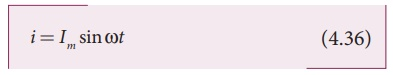Home | | Physics 12th Std | Alternating Current (AC)

# Alternating Current (AC)An alternating voltage is the voltage which changes polarity at regular intervals of time and the direction of the resulting alternating current also changes accordingly.

ALTERNATING CURRENT

Introduction

In section (AC GENERATOR), we have seen that when the orientation of the coil with the magnetic field is changed, an alternating emf is induced and hence an alternating current flows in the closed circuit. An alternating voltage is the voltage which changes polarity at regular intervals of time and the direction of the resulting alternating current also changes accordingly.

In the Figure 4.39(a), an alternating voltage source is connected to a resistor R in which the upper terminal of the source is positive and lower terminal negative at an instant. Therefore, the current flows in clockwise direction. After a short time, the polarities of the source are reversed so that current now flows in anti-clockwise direction (Figure 4.39(b)). This current which flows in alternate directions in the circuit is called alternating current.Sinusoidal alternating voltage

If the waveform of alternating voltage is a sine wave, then it is known as sinusoidal alternating voltage which is given by the relation.where Ď… is the instantaneous value of alternating voltage; Vm is the maximum value (amplitude) and Ď‰ is the angular frequency of the alternating voltage. When sinusoidal alternating voltage is applied to a closed circuit, the resulting alternating current is also sinusoidal in nature and its relation iswhere Im is the maximum value (amplitude) of the alternating current. The direction of sinusoidal voltage or current is reversed after every half-cycle and its magnitude is also changing continuously as shown in Figure 4.40.Tags : Introduction, Definition, Formula , 12th Physics : Electromagnetic Induction and Alternating Current
Study Material, Lecturing Notes, Assignment, Reference, Wiki description explanation, brief detail
12th Physics : Electromagnetic Induction and Alternating Current : Alternating Current (AC) | Introduction, Definition, Formula